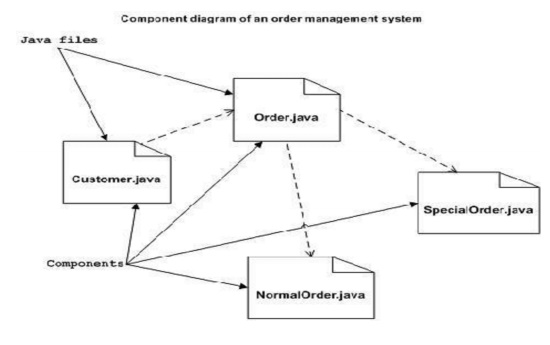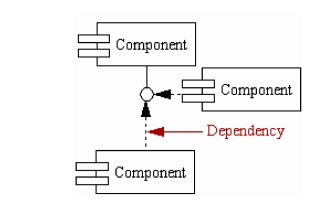Home | | Object Oriented Analysis and Design | UML Component Diagram

# UML Component Diagram

Component diagrams are different in terms of nature and behaviour. Component diagrams are used to model physical aspects of a system.

UML Component Diagram:

1. Overview:

       Component diagrams are different in terms of nature and behaviour. Component diagrams are used to model physical aspects of a system. Now the question is what are these physical aspects? Physical aspects are the elements like executables, libraries, files, documents etc which resides in a node.

       So component diagrams are used to visualize the organization and relationships among components in a system. These diagrams are also used to make executable systems.

2. Purpose:

       Component diagram is a special kind of diagram in UML. The purpose is also different from all other diagrams discussed so far. It does not describe the functionality of the system but it describes the components used to make those functionalities. So from that point component diagrams are used to visualize the physical components in a system. These components are libraries, packages, files etc.

       Component diagrams can also be described as a static implementation view of a system. Static implementation represents the organization of the components at a particular moment. A single component diagram cannot represent the entire system but a collection of diagrams are used to represent the whole. So the purpose of the component diagram can be summarized as:

1.       Visualize the components of a system.

2.       Construct executables by using forward and reverse engineering.

3.       Describe the organization and relationships of the components.

3. How to draw Component Diagram?

       Component diagrams are used to describe the physical artifacts of a system. This artifact includes files, executables, libraries etc. So the purpose of this diagram is different, Component diagrams are used during the implementation phase of an application. But it is prepared well in advance to visualize the implementation details.

       Initially the system is designed using different UML diagrams and then when the artifacts are ready component diagrams are used to get an idea of the implementation. This diagram is very important because without it the application cannot be implemented efficiently.

       A well prepared component diagram is also important for other aspects like application performance, maintenance etc. So before drawing a component diagram the following artifacts are to be identified clearly:

1.       Files used in the system.

2.       Libraries and other artifacts relevant to the application.

3.       Relationships among the artifacts.

Now after identifying the artifacts the following points needs to be followed:

1.       Use a meaningful name to identify the component for which the diagram is to be drawn.

2.       Prepare a mental layout before producing using tools.

3.       Use notes for clarifying important points.

       The following is a component diagram for order management system. Here the artifacts are files. So the diagram shows the files in the application and their relationships.

       In actual the component diagram also contains dlls, libraries, folders etc. In the following diagram four files are identified and their relationships are produced. Component diagram cannot be matched directly with other UML diagrams discussed so far. Because it is drawn for completely different purpose.

       So the following component diagram has been drawn considering all the points mentioned above:4. Where to use Component Diagrams?

       We have already described that component diagrams are used to visualize the static implementation view of a system. Component diagrams are special type of UML diagrams used for different purposes.

       These diagrams show the physical components of a system. To clarify it, we can say that component diagrams describe the organization of the components in a system.

       Organization can be further described as the location of the components in a system. These components are organized in a special way to meet the system requirements. As we have already discussed those components are libraries, files, executables etc. Now before implementing the application these components are to be organized.

       This component organization is also designed separately as a part of project execution. Component diagrams are very important from implementation perspective. So the implementation team of an application should have a proper knowledge of the component details. Now the usage of component diagrams can be described as:

1.       Model the components of a system.

2.       Model database schema.

3.       Model executables of an application.

4.       Model system's source code.

5. Basic Component Diagram Symbols and Notations

Component

A component is a physical building block of the system. It is represented as a rectangle with tabs. Learn how to resize grouped objects like components.Interface

An interface describes a group of operations used or created by components.Dependencies

Draw dependencies among components using dashed arrows. Learn about line styles in SmartDraw.6. Elements of a component diagram:

Rectangle: A single component is described using a rectangle and having the component‟s name inside it. <<Component>>Component Name

Additional compartments: Additional compartments are stacked below the component name. Interfaces provided/required: Another compartment exists for displaying the interface provided and required by the component.Subsystem: A subsystem is represented using a rectangle with stereotype <>subsystem name.

Study Material, Lecturing Notes, Assignment, Reference, Wiki description explanation, brief detail
Object Oriented Analysis and Design : UML Component Diagram |